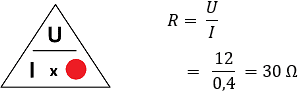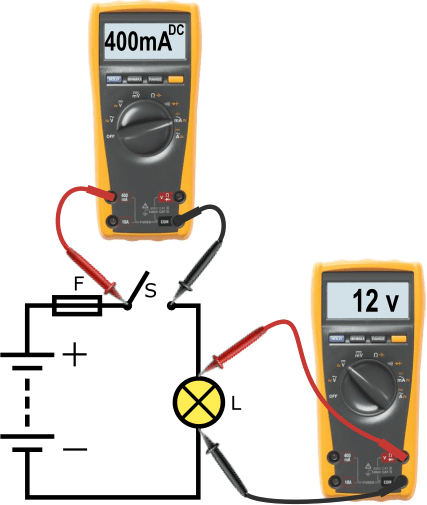# Ohm's law

Subjects:

• Introduction Ohm's Law
• Calculate voltage
• Calculate current
• Calculate resistance

Introduction Ohm's Law:
Voltage, current and resistance depend on each other. If the voltage increases with a constant resistance, the current also increases. If the resistance increases and the voltage remains the same, the current decreases. Using a formula from “Ohm's Law” one of these three factors can be calculated if two are known.

The three symbols can be placed in a triangle. On this page calculating with Ohm's Law is made clear with a number of examples.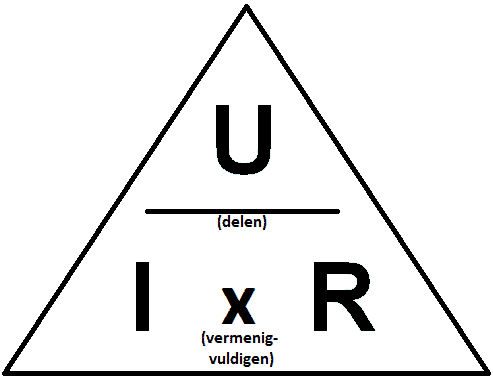The following table shows the letters, quantities, units and symbols of voltage, current and resistance. It is important to keep or memorize this table.

On the page: "basic electronics” explains the basics.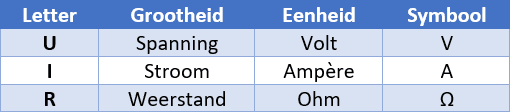The moment we know the current (I) and resistance (R) in a circuit, we can calculate the voltage (U). We take the triangle and cover the letter of what we want to calculate. In this case the U. What remains is: I * R. By multiplying the current by the resistance, we get the voltage.

The same goes for the current: when covering the I, U / R remains. By dividing the voltage by the resistance value, we get the current.

Finally, we can also calculate the resistance. Capping the R gives: U / I. Dividing the current by the voltage gives the resistance value.

In the following paragraphs there are three examples in which we calculate with the triangle of Ohm's Law.

Calculate voltage:
In this diagram, the multimeter is connected in series with the lamp. To do this, the switch was opened and measurements were taken across the switch. The multimeter is set to mA. See the page “basic electronics” for more information on measuring current.

We measure a current of 500 mA and the resistance value of the lamp is known: it is 28 ohms. Using Ohm's Law we calculate the voltage of the lamp. We cover the U and see that we have to multiply I by R to get the value U.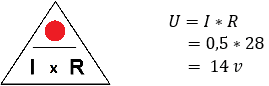We assume that there are no losses (ie interference-free). In that case, the battery voltage is 14 volts and the voltage across the lamp is 14 volts. The lamp uses the 14 volts to burn.

Calculate current:
The switch is closed and the lamp is on. The voltage is measured in parallel across the lamp with the voltmeter. The voltage is 13 volts. The resistance value is again known and is 20 ohms.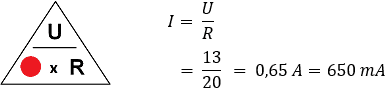The current of 650 milliamps runs through the entire circuit and is not consumed.